# Intro to Pandas: How to Add, Rename, and Remove Columns in Pandas

To make sure your DataFrame contains only the data that you want use in your project, you can add columns and remove columns from a DataFrame.
By Boris Delovski • Updated on Nov 15, 2022<< Read previous article in series: Intro to Pandas: What is a Pandas DataFrame and How to Create One

You can do a lot of different things with data that is stored inside a DataFrame. However, performing those transformations is not the first thing you do when working on a project. Typically, you first need to make sure that your DataFrame contains only the data that you want use in your project. You can do this by adding columns to a DataFrame, removing columns from a DataFrame, adding rows to a DataFrame, removing rows from a DataFrame, and by combining multiple DataFrames.

All of the aforementioned operations are extremely easy to perform, and usually boil down to using a single function. In this article I will focus on working with columns within a Pandas DataFrame. Working with rows and combining DataFrames will be covered in the subsequent article of this series.

Article continues below

## How to Add Columns to a Pandas DataFrame

Adding a column to a Pandas DataFrame is probably the easiest operation you can perform with a DataFrame. It actually doesn't require you to use any function, you only need to define the column name and the data that you want to store in that column.

To demonstrate with an example, let's first create a simple DataFrame and then let's add a column to it.

I will create a DataFrame that contains the starting character of a country name inside the Letter column, and the country name itself in the Country column:

``country_df = pd.DataFrame({"Letter": ["a", "b", "c"], "Country": ["Andorra", "Belgium", "Croatia"]})``

The DataFrame that I just created looks like this: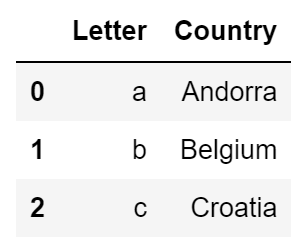Image Source: A screenshot of a Pandas Dataframe, Edlitera

If I want to add a new column to that DataFrame, I just need to reference the DataFrame itself,  add the name of the new column in the square brackets, and finally supply the data that I want to store inside of the new column.

For example, let's add a new column called GDP to our DataFrame, and make sure that the value of all the rows in it is equal to 0:

``country_df["GDP"] = 0``

This is all I need to do to add a new column to a DataFrame. After running the code above, my DataFrame will look like this: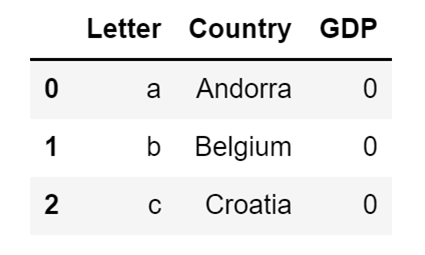Image Source: A screenshot of a Pandas DataFrame with the an added column, Edlitera

As you can see, the whole process is very simple.

One thing you need to be careful about here is that if you reference a column that already exists, you will overwrite the data that is stored inside of it because of a very simple reason: DataFrame columns are Pandas Series objects. This means that adding a column to a Pandas DataFrame works almost like defining which Pandas Series you want to have stored under which column name in our DataFrame.

If you try to store a Pandas Series object under some column name that doesn't exist, then Pandas will create a new column with that column name. However, if a column name already exists, it will be basically tell Pandas to replace the Series stored under a particular column name with another Series.

Let's demonstrate this by creating a Pandas Series object and storing it in the variable GDP_series. That Series will contains the values 0, 1, and 2. I will afterward assign it to the GDP column of my DataFrame:

``````GDP_series = pd.Series([0, 1, 2])

country_df["GDP"] =  GDP_series``````

The code above will replace the values in my GDP column, which were previously all 0, with 0, 1, and 2. The new version of our DataFrame will then look like this: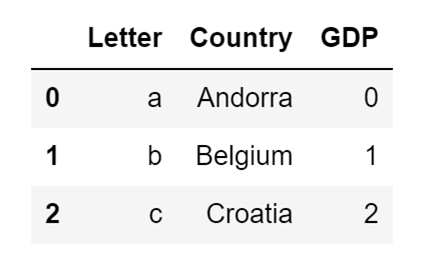Image Source: A screenshot example of data in a DataFrame being replaced with a Pandas Series object, Edlitera

## How to Rename Pandas DataFrame Columns

If you want to add a new column to a DataFrame that already contains a column with that same name, the easiest way of making sure that your data will not be overwritten is to rename the column that is already inside the DataFrame

To do that, you use the Pandas rename() method. You just input a dictionary that contains old column names as the keys, and the new column names as the values. So if I want to, for example, rename the Country column so that its new name is Country/Territory, I will use the following code:

``country_df.rename(columns={"Country": "Country/Territory"}, inplace=True)``

The result I get after running the code above is a DataFrame that looks like this: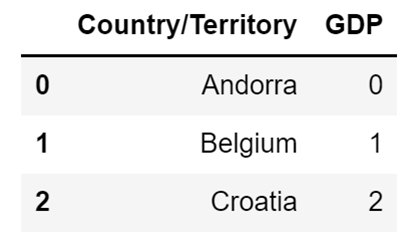Image Source: A screenshot example of renaming a DataFrame column without replacing the data, Edlitera

You might notice an extra argument if you carefully analyze the code I used to rename my column: the in-place argument which I defined as True. If I don't do this, Pandas won't rename my column, but will instead return a display that shows how my DataFrame would look like if I were to actually change the name of my column.

This is something that is not exclusive to the renaming of columns in Pandas. It is something that applies to many different operations (even to dropping columns as I will demonstrate in a few moments). It allows you to see what the modified version of your DataFrame would look like before you modify it.

To demonstrate, let's say that I want to see how my DataFrame would look like if I were to rename my GDP column into gdp. To do that I can use the rename() method without specifying the inplace argument as True:

``country_df.rename(columns={"GDP": "gdp"})``

The code above will automatically display the following result: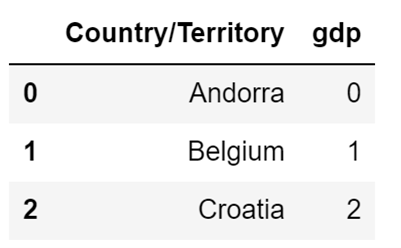Image Source: A screenshot of renaming a column without specifying the inplace argument as True, Edlitera

However, my DataFrame didn't change. If I look at it by calling its name, I will see that my DataFrame still looks like this:Image Source: A screenshot of the unchanged DataFrame when the name is called without the inplace argument, Edlitrea

Therefore, to change my DataFrame I must use the inplace argument.

## How to Remove Columns From a DataFrame

To remove a column from a DataFrame, you use the drop() method.

Again, similarly to how the rename() method works, you need to specify the value of the inplace argument as True if you want to modify our DataFrame.

If you don't use that argument the drop() method will just display what would be the final result, but it won't modify your DataFrame.

Let's create an example DataFrame with more than two columns:

``````df = pd.DataFrame({
"Letter": ["a", "b", "c"],
"Country": ["Andorra", "Belgium", "Croatia"],
"Capital": ["Andorra la Vella", "Brussels", "Zagreb"],
"GDP per capita": [41873, 42787, 12984]
})``````

The code above will create the following DataFrame:Image Source: A screenshot of a Pandas DataFrame with more than two columns, Edlitera

If I want to see how my DataFrame would look like if I removed the Letter and GDP per capita columns, I just need to input a list of column names to the columns argument of my drop() method:

``df.drop(columns=["Letter", "GDP per capita"])``

The code above will result in the following display: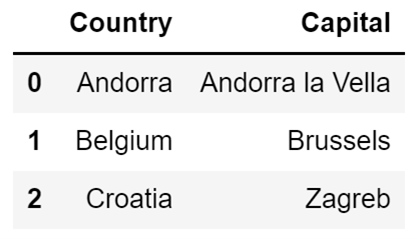Image Source: A screenshot example of removing a Pandas DataFrame column using the drop method, Edlitera

But as mentioned previously, because I didn't set the inplace argument to True, my DataFrame wasn't modified.

If we do want to modify it, I just need to set the inplace argument to True:

``df.drop(columns=["Letter", "GDP per capita"], inplace=True)``

If I check the DataFrame after running the code above, it will only contain the Country and Capital columns.

In this article, I covered the basics of adding and removing data from Pandas DataFrames. I demonstrated how to add and remove columns from a Pandas DataFrame, and I even demonstrated how to rename columns. These are some of the most common operations you will perform at the beginning of your data processing pipeline, alongside manipulating columns (which I will cover in the following article).

Only when you remove all useless data from your DataFrame can you actually focus on analyzing data and drawing conclusions, because using unneeded data is counterproductive. If you combine the knowledge from the previous articles in the series on Pandas with what you learned in this article, and what you will learn by reading the following articles in this Pandas article series, you’ll be completely prepared to create DataFrames for processing and will improve the results of your analysis

• Read next in the series: Intro to Pandas: How to Create and Analyze Basic Pandas DataFrames >># Boris Delovski

Boris is a data science trainer and consultant who is passionate about sharing his knowledge with others.

Before Edlitera, Boris applied his skills in several industries, including neuroimaging and metallurgy, using data science and deep learning to analyze images.